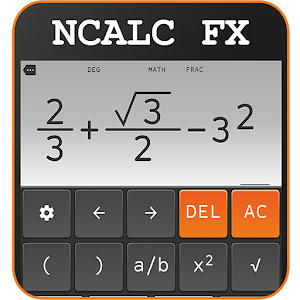Education

# School Scientific calculator casio fx 570 es plusMr Duy

2.2.6

02. 09. 2018

N/A

#### Compatible:

4.0 and up
This is all in one calculator, full mathematics function, working offline. A scientific calculator supports most of the features of fx 500, fx500, JX570ms, 570vnplus, 991es plus, 991ms, fx570esplus, fx-82 or TI-30XB, fx 4500, 82ms, 82es, fx-82AU PLUS II, 100AU, fx 4500pa, 991ex, fx 260.

Natural display
Natural Input allowing you to enter equations as you'd write them on paper, using fractions, roots, exponents and more. It's called a "natural display" and is a common feature on scientific calculators like the Casio fx 570 991 es plus!.

Scroll feature
Swipe on the keyboard to move the cursor up and down, left and right. Touch to edit expression.

• Solve equation (Shift solve)
• CALC function (with memory function, 8 variable)
• Derivative function
• Integrate function
• Base N calculation (Calculator binary, decimal, hexadecimal, octal)
• Table generator: generate table base on expression.
• Solve system equation (two and three unknown variable)
• Calculate with fraction result (fraction calculator)
• Calculate with big numbers
• Complex number
• Matrix calculator with full feature of algebra calculator
• Percent % calculator
• logarithms calculator (log base n)

Output type
• Fraction (1/2)
• Mixed fraction (4/3 = 1 3/4)
• Decimal (1.23123)
• Repeat decimal (1.23232323 = 1.(23))
• Degree/Minus/Second
• Polar coordinate (Pol, Rec function)

Decimal Notation
Normal, Engineering, Engineering (SI), Science, Fixed

More feature
• Automatic calculate: see result as you typing (turn off in setting)
• Vibrate, vibrate strength
• Play sound when click sound button
• Decimal formatter
• Calculation history
• Show variable value
• Two layout: full keyboard and compact keyboard
• Change font family
• Change theme
• Change font size
• Copy, paste expression (long click to copy)